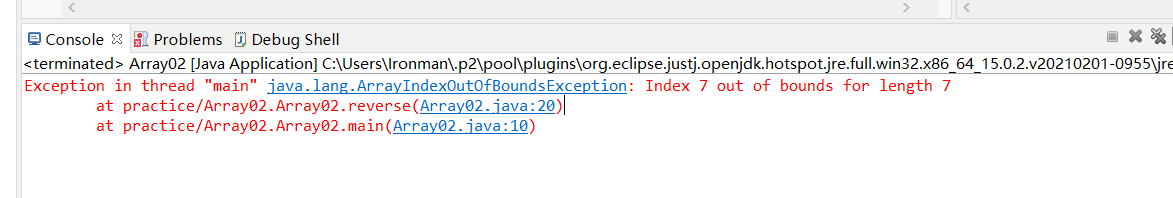2021-03-03 23:31

# 救救萌新！！救救孩子，简单的翻转数组问题。package Array02;

public class Array02 {

public static void main(String[] args) {

//普通for循环打印数组
int[] Aarrays = {1,2,3,4,5,6,7};

reverse(Aarrays);
//for each打印数组（没有下标，不好取数操作）
}

//翻转数组
public static int[] reverse(int[] arrays) {
int [] result = new int[arrays.length];

for(int i = 0 , j = result.length;i < j;i++,j--) {
result[i]=arrays[j];
System.out.print(arrays[i]+" ");

}

return result;

}

//计算数组长度的方法
public static void Printarrays(int[] printarrays) {
for(int i=0;i<printarrays.length;i++) {
System.out.print(printarrays[i]+" ");
}
}
}

• 点赞
• 写回答
• 关注问题
• 收藏
• 复制链接分享
• 邀请回答

#### 3条回答

• ``````public class Array02 {

public static void main(String[] args) {
//普通for循环打印数组
int[] Aarrays = {1, 2, 3, 4, 5, 6, 7};
int[] result = reverse(Aarrays);
//for each打印数组（没有下标，不好取数操作）
Printarrays(result);
}

//翻转数组
public static int[] reverse(int[] arrays) {
int[] result = new int[arrays.length];
for (int i = 0; i < arrays.length; i++) {
result[i] = arrays[arrays.length - i - 1];
}
return result;
}

//计算数组长度的方法
public static void Printarrays(int[] printarrays) {
for (int i = 0; i < printarrays.length; i++) {
System.out.print(printarrays[i] + " ");
}
}
}``````
点赞 评论 复制链接分享
•  for(int i = 0 , j = result.length;i < j;i++,j--) 这句话出的问题。result.length=7,是数组的长度，但是数组下标是从0开始的，也就是说 你的下面那句result[i]=arrays[j];其中j=7就数组越界了，j = result.length-1才对。

点赞 评论 复制链接分享
• 修改了reverse方法，因为int[]是对象传递，所以可以用void代替

希望回答对你有帮助

``````public static void main(String[] args) {

//普通for循环打印数组
int[] Aarrays = {1,2,3,9,5,6,7};

reverse(Aarrays);
//for each打印数组（没有下标，不好取数操作）

// 打印反转的结果
for (int i = 0; i<Aarrays.length; i++){
System.out.print(Aarrays[i]);
}
}

//翻转数组
public static void reverse(int[] arrays) {
// 计算需要转换的次数
int size = arrays.length / 2;
int a;
for(int i = 0, j = arrays.length -1 ; i<size; i++, j--) {
a = arrays[i];
// 数据替换
arrays[i] = arrays[j];
arrays[j] = a;
}
}

//计算数组长度的方法
public static void Printarrays(int[] printarrays) {
for(int i=0;i<printarrays.length;i++) {
System.out.print(printarrays[i]+" ");
}
}``````
点赞 评论 复制链接分享